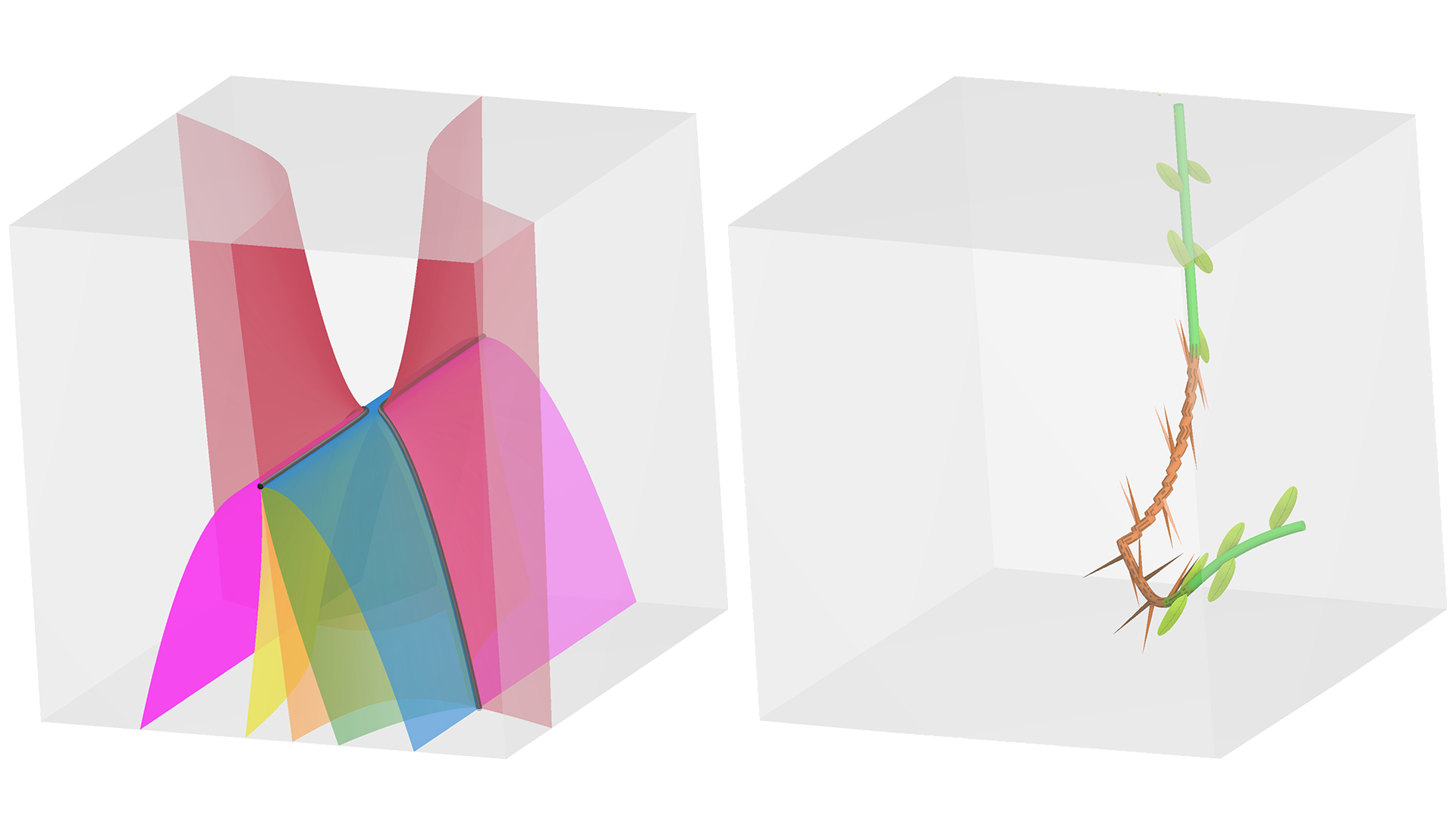# Feature Curves and Surfaces of 3D Asymmetric Tensor Fields

### Shih-Hsuan Hung, Yue Zhang, Harry Yeh, Eugene Zhang

#####  View presentation:2021-10-26T15:45:00Z GMT-0600 Change your timezone on the schedule page 2021-10-26T15:45:00ZThis paper introduces topological feature curves and surfaces for the analysis of 3D asymmetric tensor fields, such as the velocity gradient tensor of the Lorenz attractor (left). Note all these surfaces intersect at the triple degenerate curves. Furthermore, this analysis leads to an eigenvalue space for 3D asymmetric tensor fields. To enable a holistic view of the eigenvectors and dual-eigenvectors, this paper proposes an augmented hyperstreamline following one eigenvector field as a tree stem with attached thorns and leaves to show the other eigenvectors or dual-eigenvectors (right).
##### Abstract

3D asymmetric tensor fields have found many applications in science and engineering domains, such as fluid dynamics and solid mechanics. 3D asymmetric tensors can have complex eigenvalues, which makes their analysis and visualization more challenging than 3D symmetric tensors. Existing research in tensor field visualization focuses on 2D asymmetric tensor fields and 3D symmetric tensor fields. In this paper, we address the analysis and visualization of 3D asymmetric tensor fields. We introduce six topological surfaces and one topological curve, which lead to an eigenvalue space based on the tensor mode that we define. In addition, we identify several non-topological feature surfaces that are nonetheless physically important. Included in our analysis are the realizations that triple degenerate tensors are structurally stable and form curves, unlike the case for 3D symmetric tensors fields. Furthermore, there are two different ways of measuring the relative strengths of rotation and angular deformation in the tensor fields, unlike the case for 2D asymmetric tensor fields. We extract these feature surfaces using the A-patches algorithm. However, since three of our feature surfaces are quadratic, we develop a method to extract quadratic surfaces at any given accuracy. To facilitate the analysis of eigenvector fields, we visualize a hyperstreamline as a tree stem with the other two eigenvectors represented as thorns in the real domain or the dual-eigenvectors as leaves in the complex domain. To demonstrate the effectiveness of our analysis and visualization, we apply our approach to datasets from solid mechanics and fluid dynamics.